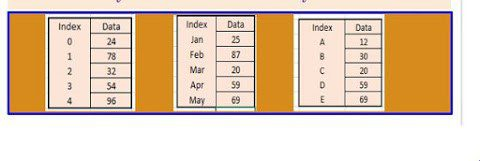In this article, Data handling using Pandas-I you will learn about the Python Pandas data structure series.

Topics Covered

## Introduction to Python Libraries

Python libraries are in-built python modules that allow performing system-related operations, IO operations, data analysis and some other standard operations. Pandas library is used for data analysis.

## Introduction to Data handling using Pandas-I

Important points for pandas:

• Pandas word derived from PANel Data System.
• It becomes popular for data analysis.
• It provides highly optimized performance with back-end source code purely written in C or Python.
• It makes a simple and easy process for data analysis.

Pandas offer two basic data structures:

1. Series
2. DataFrame

To work with pandas import the pandas library and create one object like this:

``import pandas as pd``

## Data handling using Pandas-I Series

Series is an important data structure of pandas. It represents a one-dimensional array, containing an array of data. It can be any type of NumPy data. Basically series has two main components:

1. An Array
2. An index associated with an array

Example:

Series() function is used to create a series in Pandas.

Example:

``````import pandas as pd
ser1=pd.Series()
``````

An empty panda series has float64 data type.

### Creating non-empty series

In non-empty series data and index will be supplied while creating series. Here data can be one of these data types:

1. A python sequence
2. An ndarray
3. A dictionary
4. A scalar value

### Creating series with a python sequence (Data handling using Pandas-I)

Range function is used to generate a series with python pandas.

In the above screenshot, a series is created with float numbers.

### Creating Series with ndarray (Data handling using Pandas-I)

Creating a series from ndarray named nda. An array of an odd number between 1 to create through the range.

### Creating series with a dictionary

Crating series from Dictionary object and stored first three days of the week in series.

### Creating series with a scalar value

Series created with scalar value 5.

### Task 2 Specifying NaN values in the series

Specified NaN at the index 1.

### Task 3 creating series and specifying index

In the above example, two lists were created for train numbers and train names. Train no list assigned as data and train name assigned as indexes.

### Task 4 Creating series using arithmetic operation

In this example, series is created with a * 3 as data.

## Data handling using Pandas-I Common Series attributes

### Task 5 Common series attribute Example

Watch this video to understand the practical aspects:

## Task 6 Accessing elements from series

In above screenshot, I have accessed elements by using their index value such as ser and ser. For accessing all the values using indexes you can use for loop.

## Task 7 Modifying series elements

In above code, I have changed the element value with a scalar value. In python, series objects are valued mutable i.e. values can be changed but size immutable i.e. can’t be changed.

## Task 9 head() and tail() function in series (Data handling using Pandas-I)

The head() function displays n number of elements from the top in the series. In the above example, I have accessed top 3 elements. If no value is passed in the parameter then by default it will display 5 elements from the top. Similarly, the tail function will work and display n number of elements from the bottom.

### Task 10 Vector and arithmetic operations on series

Here I have used different vector operations and operators to perform various tasks on series.

Watch this video for practical understanding:

## Task 11 reindex() and drop() methods – (Data handling using Pandas-I)

reindex() : Create a similar object but with a different order of same indexes.